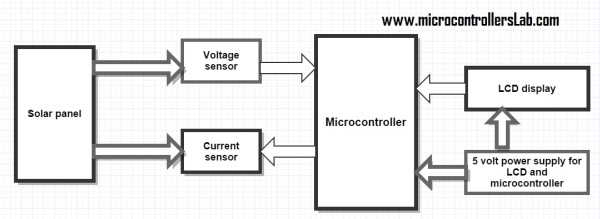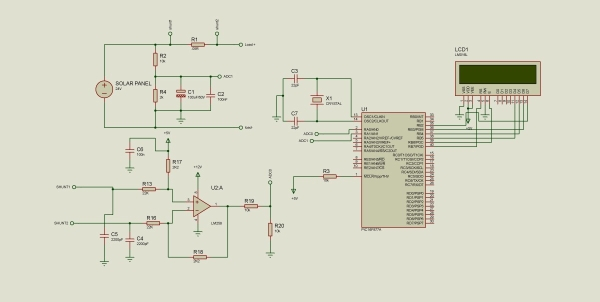# Solar energy measurement using pic microcontroller

### Solar energy measurement:

This project is designed to measured energy of solar panels. In this project you will get a idea how to meausre solar energy using different sensors and pic microcontroller. Followings are the main parts used in this project :

• Current sensor
• voltage sensor
• PIC16F877A microcontroller
• LCD display
• Power supplyBlock diagram of solar energy measurement system:

At the right side of diagram is solar panel which energy you wan to measure. Voltage sensor is used to measure voltage of solar panel. Current sensor is used to measure current flowing to load from solar panel. As we know, solar panels are dc power sources. So output voltage and current solar panel is DC. So we can easily measure solar power by using dc power formula. I will discuss it later. Liquid crystal display  is used to display value of current, voltage and power of solar panel. 5 volt dc power is used to provide operating voltages to microcontroller and liquid crystal display. You can either design dc power supply from ac power source or you can use battery to provide constant 5 volt to microcontroller and LCD.

### Circuit diagram of solar energy measurement system:

Circuit diagram of solar energy meter is given below. I will explain each and every step of this article in video in last part of this article.In above given circuit diagram, voltage divider is used to divide voltage to lower than 5 volt. Because microcontroller can not read voltage more than 5 volt. Therefor voltage divider is used to lower voltage less than 5 volt. Polar and non polar capacitors are used to remove harmonics and to provide constant voltage to adc pin of microcontroller. I will explain about analog to digital converter later or either i will post separate article on it. Polar capacitor is used to avoid voltage fluctuation and non polar capacitor is used remove harmonics and it will stop harmonics to read to microcontroller which may damage microcontroller.

READ  Digital Thermometer using a PIC Microcontroller and DS18B20

For more detail: Solar energy measurement using pic microcontroller

#### Current Project / Post can also be found using:

• pic microcontroller solar measurement
• solar measurement using pic microcontroller pp
• how the motor is used to control the solar panel using pic microcontroller
• Pic controller based solar energy measurements